Skip to main content Accessibility help
Home
Hostname: page-component-684bc48f8b-vgwqb Total loading time: 0.692 Render date: 2021-04-11T11:03:17.430Z Has data issue: true Feature Flags: { "shouldUseShareProductTool": true, "shouldUseHypothesis": true, "isUnsiloEnabled": true, "metricsAbstractViews": false, "figures": false, "newCiteModal": false, "newCitedByModal": true }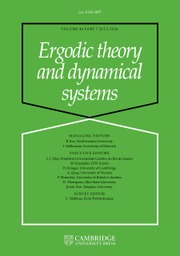Ergodic Theory and Dynamical Systems

# The sets of Dirichlet non-improvable numbers versus well-approximable numbers

Published online by Cambridge University Press:  27 June 2019

Corresponding
E-mail address:

## Abstract

Let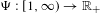$\unicode[STIX]{x1D6F9}:[1,\infty )\rightarrow \mathbb{R}_{+}$ be a non-decreasing function,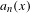$a_{n}(x)$ the$n$ th partial quotient of$x$ and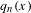$q_{n}(x)$ the denominator of the$n$ th convergent. The set of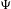$\unicode[STIX]{x1D6F9}$ -Dirichlet non-improvable numbers,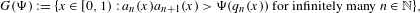$$\begin{eqnarray}G(\unicode[STIX]{x1D6F9}):=\{x\in [0,1):a_{n}(x)a_{n+1}(x)>\unicode[STIX]{x1D6F9}(q_{n}(x))\text{ for infinitely many }n\in \mathbb{N}\},\end{eqnarray}$$
is related with the classical set of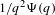$1/q^{2}\unicode[STIX]{x1D6F9}(q)$ -approximable numbers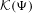${\mathcal{K}}(\unicode[STIX]{x1D6F9})$ in the sense that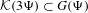${\mathcal{K}}(3\unicode[STIX]{x1D6F9})\subset G(\unicode[STIX]{x1D6F9})$ . Both of these sets enjoy the same$s$ -dimensional Hausdorff measure criterion for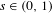$s\in (0,1)$ . We prove that the set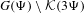$G(\unicode[STIX]{x1D6F9})\setminus {\mathcal{K}}(3\unicode[STIX]{x1D6F9})$ is uncountable by proving that its Hausdorff dimension is the same as that for the sets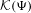${\mathcal{K}}(\unicode[STIX]{x1D6F9})$ and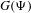$G(\unicode[STIX]{x1D6F9})$ . This gives an affirmative answer to a question raised by Hussain et al [Hausdorff measure of sets of Dirichlet non-improvable numbers. Mathematika 64(2) (2018), 502–518].

## MSC classification

Type
Original Article
Information
Ergodic Theory and Dynamical Systems , December 2020 , pp. 3217 - 3235
Copyright
© Cambridge University Press, 2019

## Access options

Get access to the full version of this content by using one of the access options below.

## References

Beresnevich, V., Dickinson, D. and Velani, S.. Measure theoretic laws for lim sup sets. Mem. Amer. Math. Soc. 179(846) (2006).Google Scholar
Bugeaud, Y.. Exponents of Diophantine approximation. Dynamics and Analytic Number Theory (London Mathematical Society Lecture Note Series, 437). Cambridge University Press, Cambridge, 2016, pp. 96135.CrossRefGoogle Scholar
Cassels, J. W. S.. An Introduction to Diophantine Approximation (Cambridge Tracts in Mathematics and Mathematical Physics, 45). Cambridge University Press, Cambridge, 1957.Google Scholar
Davenport, H. and Schmidt, W. M.. Dirichlet’s theorem on diophantine approximation. Symposia Mathematica, Vol. IV (INDAM, Rome, 1968/69). Academic Press, London, 1970, pp. 113132.Google Scholar
Diviš, B.. A analog to the Lagrange numbers. J. Number Theory 4 (1972), 274285.CrossRefGoogle Scholar
Diviš, B. and Novák, B.. A remark on the theory of Diophantine approximations. Comment. Math. Univ. Carolin. 12 (1971), 127141.Google Scholar
Dodson, M. M.. Star bodies and Diophantine approximation. J. Lond. Math. Soc. (2) 44(1) (1991), 18.Google Scholar
Dodson, M. M.. Hausdorff dimension, lower order and Khintchine’s theorem in metric Diophantine approximation. J. Reine Angew. Math. 432 (1992), 6976.Google Scholar
Falconer, K.. Fractal Geometry, 3rd edn. Wiley, Chichester, 2014.Google Scholar
Haas, A.. The relative growth rate for partial quotients. New York J. Math. 14 (2008), 139143.Google Scholar
Huang, L. and Wu, J.. Uniformly non-improvable Dirichlet set via continued fractions. Proc. Amer. Math. Soc. (2019), to appear, doi:10.1090/proc/14587.CrossRefGoogle Scholar
Hussain, M. and Simmons, D.. A general principle for Hausdorff measure. Proc. Amer. Math. Soc., to appear, doi:10.1090/proc/14539.Google Scholar
Hussain, M., Kleinbock, D., Wadleigh, N. and Wang, B.-W.. Hausdorff measure of sets of Dirichlet non-improvable numbers. Mathematika 64(2) (2018), 502518.CrossRefGoogle Scholar
Khintchine, A. Ya.. Continued Fractions. P. Noordhoff, Groningen, 1963, translated by Peter Wynn.Google Scholar
Kim, D. H. and Liao, L.. Dirichlet uniformly well-approximated numbers. Int. Math. Res. Not., to appear. Preprint, 2015, arXiv:1508.00520.Google Scholar
Kleinbock, D. and Wadleigh, N.. A zero-one law for improvements to Dirichlet’s theorem. Proc. Amer. Math. Soc. 146(5) (2018), 18331844.CrossRefGoogle Scholar
Kristensen, S.. Metric Diophantine approximation—from continued fractions to fractals. Diophantine Analysis: Course Notes from a summer School (Trends Math.). Ed. Steuding, J.. Birkhäuser/Springer, Cham, 2016, pp. 61127.CrossRefGoogle Scholar
Legendre, A.-M.. Essai sur la théorie des nombres (Essay on Number Theory) (Cambridge Library Collection). Reprint of the second (1808) edn. Cambridge University Press, Cambridge, 2009, (French).CrossRefGoogle Scholar
Daniel Mauldin, R. and Urbański, M.. Conformal iterated function systems with applications to the geometry of continued fractions. Trans. Amer. Math. Soc. 351(12) (1999), 49955025.CrossRefGoogle Scholar
Waldschmidt, M.. Recent Advances in Diophantine Approximation (Number Theory, Analysis and Geometry). Springer, New York, 2012, pp. 659704.Google Scholar
Wang, B.-W. and Wu, J.. Hausdorff dimension of certain sets arising in continued fraction expansions. Adv. Math. 218(5) (2008), 13191339.CrossRefGoogle Scholar

### Full text views

Full text views reflects PDF downloads, PDFs sent to Google Drive, Dropbox and Kindle and HTML full text views.

Total number of HTML views: 0
Total number of PDF views: 159 *
View data table for this chart

* Views captured on Cambridge Core between 27th June 2019 - 11th April 2021. This data will be updated every 24 hours.

# Send article to Kindle

To send this article to your Kindle, first ensure no-reply@cambridge.org is added to your Approved Personal Document E-mail List under your Personal Document Settings on the Manage Your Content and Devices page of your Amazon account. Then enter the ‘name’ part of your Kindle email address below. Find out more about sending to your Kindle. Find out more about sending to your Kindle.

Note you can select to send to either the @free.kindle.com or @kindle.com variations. ‘@free.kindle.com’ emails are free but can only be sent to your device when it is connected to wi-fi. ‘@kindle.com’ emails can be delivered even when you are not connected to wi-fi, but note that service fees apply.

Find out more about the Kindle Personal Document Service.

The sets of Dirichlet non-improvable numbers versus well-approximable numbers
Available formats
×

# Send article to Dropbox

To send this article to your Dropbox account, please select one or more formats and confirm that you agree to abide by our usage policies. If this is the first time you use this feature, you will be asked to authorise Cambridge Core to connect with your <service> account. Find out more about sending content to Dropbox.

The sets of Dirichlet non-improvable numbers versus well-approximable numbers
Available formats
×

# Send article to Google Drive

To send this article to your Google Drive account, please select one or more formats and confirm that you agree to abide by our usage policies. If this is the first time you use this feature, you will be asked to authorise Cambridge Core to connect with your <service> account. Find out more about sending content to Google Drive.

The sets of Dirichlet non-improvable numbers versus well-approximable numbers
Available formats
×
×

#### Conflicting interests

Do you have any conflicting interests? *